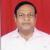true

Take Class 10 Tuition from the Best Tutors

•Affordable fees
•1-1 or Group class
•Flexible Timings
•Verified Tutors

Search in

# Charge (electric charge)Sudhansu Bhushan Roy
15/04/2018 00

Charge (electric charge)

In physics, charge, also known as electric charge, electrical charge, or electrostatic charge and symbolized q, is a characteristic of a unit of matter that expresses the extent to which it has more or fewer electrons than protons. In atoms, the electron carries a negative elementary or unit charge; the proton carries a positive charge. The two types of charge are equal and opposite.

In an atom of matter, an electrical charge occurs whenever the number of protons in the nucleus differs from the number of electrons surrounding that nucleus. If there are more electrons than protons, the atom has a negative charge. If there are fewer electrons than protons, the atom has a positive charge. The amount of charge carried by an atom is always a multiple of the elementary charge, that is, the charge carried by a single electron or a single proton. A particle, atom, or object with negative charge is said to have negative electric polarity; a particle, atom, or object with positive charge is said to have positive electric polarity.

In an object comprised of many atoms, the net charge is equal to the arithmetic sum, taking polarity into account, of the charges of all the atoms taken together. In a massive sample, this can amount to a considerable quantity of elementary charges. The unit of electrical charge in the International System of Units is the coulomb (symbolized C), where 1 C is equal to approximately 6.24 x 1018 elementary charges. It is not unusual for real-world objects to hold charges of many coulombs.

An electric field, also called an electrical field or an electrostatic field, surrounds any object that has charge. The electric field strength at any given distance from an object is directly proportional to the amount of charge on the object. Near any object having a fixed electric charge, the electric field strength diminishes in proportion to the square of the distance from the object (that is, it obeys the inverse square law).

When two objects having electric charge are brought into each other's vicinity, an electrostatic force is manifested between them. (This force is not to be confused with electromotive force, also known as voltage.) If the electric charges are of the same polarity, the electrostatic force is repulsive. If the electric charges are of opposite polarity, the electrostatic force is ractive. In free space (a vacuum), if the charges on the two nearby objects in coulombs are q1 and q2 and the centers of the objects are separated by a distance r in meters, the net force F between the objects, in newtons, is given by the following formula:

F = (q1q2) / (4or2)

where o is the permittivity of free space, a physical constant, and  is the ratio of a circle's circumference to its diameter, a dimensionless mathematical constant. A positive net force is repulsive, and a negative net force is attractive. This relation is known as Coulomb's law.

0 Dislike
Follow 0## Other Lessons for You

How Do Organisms Reproduce(Notes for Exams)
How Do Organisms  Reproduce What is the importance of DNA copying in reproduction? Answer: DNA copying in reproduction is important for maintenance...The Trend of Electron Affinity in Periodic Table
The electron affinity (Eea) of a molecule or an atom or is the amount of energy spent or released when an electron is added to a neutral atom or molecule in the gaseous state to form a negative...If the earth were cubic in shape, what would happen when you walk over an edge?
If the earth were a cube, with the same mass as the more traditional ball-shaped version (which is 12,756 km or 7,926 mm in diameter), it would have twelve edges that were just over 9,300 km (5,800 mi)...Why have humans been to outer space but not to the bottom of the ocean?
The average depth of the ocean is about 4km. Some deep-sea vessels are capable of reaching this depth.The deepest point in the Ocean is the Mariana Trench at about 11km. This depth was also reached by...Hi Children, Don't be tensed while writing the answers. Read the questions 2 to 3 times. Recall the answers and write with neat handwriting from the first sentence of the first page till the last sentence...### Looking for Class 10 Tuition ?

Learn from Best Tutors on UrbanPro.

Are you a Tutor or Training Institute?

Join UrbanPro Today to find students near you

X

### Looking for Class 10 Tuition Classes?

The best tutors for Class 10 Tuition Classes are on UrbanPro

• Select the best Tutor
• Book & Attend a Free Demo
• Pay and start Learning### Take Class 10 Tuition with the Best Tutors

The best Tutors for Class 10 Tuition Classes are on UrbanPro